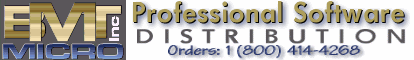the REXX Files - by Dr. Dirk Terrell

This month let's take a look at some of the formatting functions available in REXX. REXX excels at handling text files, so it should come as no surprise that there are several functions for formatting text. LEFT, RIGHT, and CENTER (or CENTRE if you prefer) are useful functions whose names imply their use.

Let's say we have some lines of text that we would like to write out to a file, with the text justified on the right. If we simply print out the strings with the SAY command, the result will look like:

```This is line 1.
This is the second line.
Line 3.```
But we would like it to look like this:
```         This is line 1.
This is the second line.
Line 3.```
The RIGHT function will do the job nicely. The calling form is:

where string is the string we are formatting, length is the desired length of the output string, and pad is the character for padding the output string. This function returns the rightmost length characters of the string. If the length of the input string is less than length, the function will pad it with the character specified in pad. (If you don't specify pad, then a space is used.)

If the string is longer than length, then the extra characters on the left will be truncated. In our application, we don't want any truncation to occur, so we need to know what value of length to use. Obviously we want it to be the size of the largest string that we have. REXX can figure that out in the blink of an eye. Just loop through all of the strings and use the LENGTH function to get the length:

```/* Find the largest string */
String.1="This is line 1."
String.2="This is the second line."
String.3="Line 3."
Max=0
Do i=1 to 3
L=Length(String.i)
If L>Max then Do
Max=L
Index=i
End
End
Say "String number" Index "was the longest with a length of" Max "characters."```
Running the above code gives the expected result:
`String number 2 was the longest with a length of 24 characters.`
Now that we know what value to use for length in our call to the RIGHT function, we use a loop to call it for each string:
```Do i=1 to 3
NewString.i=Right(String.i,Max)
Say NewString.i
End```
Note that we use the value in variable Max as the value for length. Since we did not specify pad, a space is used by default. Running the code results in:
```         This is line 1.
This is the second line.
Line 3.```
which is just what we wanted.

The LEFT function works in exactly the same way, except that it returns the leftmost length characters of a string. So, if you need to justify strings on the left, it is the function to use.

The CENTER function (or, again, CENTRE for you Brits and Canadians), will center a string. The calling form is:

where the parameters have the same meanings as before. So, if we simply replace the calls to RIGHT in the code above with calls to CENTER, we get the following result:

```    This is line 1.
This is the second line.
Line 3.```
If we wanted asterisks to be used as the pad character, we would make the calls to CENTER with the following form:

Center(String.i,Max,"*")

The output then looks like this:

```****This is line 1.*****
This is the second line.
********Line 3.*********```
Let's conclude with a formatting function for numbers. The FORMAT function is used to format strings that represent numbers. The calling form is:

Format(string, before, after, exponent_digits, exponent_trigger)

where string is the string that contains the number to be formatted, before is the number of digits to the left of the decimal point, after is the number of digits to the right of the decimal point, exponent_digits is the number of digits to be used in the exponent when exponential notation is used, and exponent_trigger is the trigger point for the function to use exponential notation (e.g. 1.0E6 instead of 1000000). So, if we have some numbers with variable numbers of digits after the decimal point and we want to round them all to 2 decimal places, we would call FORMAT with after set to 2.

For example, let's say we have the numbers 1.9865976 and 656.9769866. The following code will round them to two decimal places:

```/* Round numbers to two digits */
Number.1=1.9865976
Number.2=656.9769866
Do i=1 to 2
NewNumber.i=Format(Number.i,3,2)
Say NewNumber.i
End```
The output of the program is
```  1.99
656.98```
Notice that the value of before was set to 3 because we have a number (656.9769866) that has three digits before the decimal point. Because the other number had only one digit before the decimal point, it was padded with spaces on the left. If before is smaller than the number of digits needed, the program will stop and print out an error message. (Try running the above code with before set to 2 and you will get the "Incorrect Call to Routine" error message.) Should after be larger than the number of digits after the decimal place, the padding is done with zeroes.

LEFT, RIGHT, CENTER/CENTER, and FORMAT are useful functions when you need to control the appearance of output strings. True to the design principles of REXX, they are easy to understand, but very powerful in their capabilities.

Dr. Dirk Terrell is an astronomer at the University of Florida specializing in interacting binary stars. His hobbies include cave diving, martial arts, painting and writing OS/2 software such as HTML Wizard.

[Our Sponsor: Indelible Blue - OS/2 software and hardware solutions to customers worldwide.]